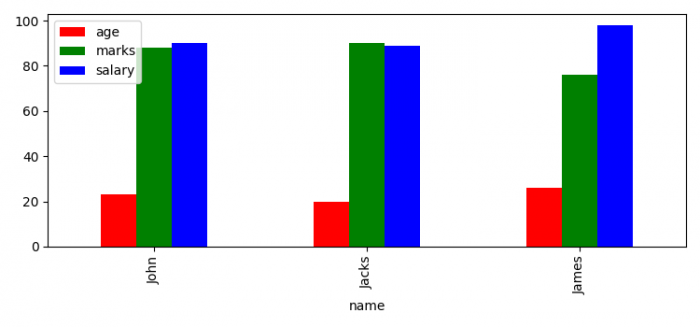# How do I make bar plots automatically cycle across different colors?

To male bar plots automatically cycle across different colors, we can take the following steps −

• Set the figure size and adjust the padding between and around the subplots.
• Set automatic cycler for different colors.
• Make a Pandas dataframe to plot the bars.
• Use plot() method with kind="bar" to plot the bars.
• To display the figure, use show() method.

## Example

import matplotlib.pyplot as plt
import pandas as pd

plt.rcParams["figure.figsize"] = [7.50, 3.50]
plt.rcParams["figure.autolayout"] = True
plt.rc('axes', prop_cycle=(plt.cycler('color', ['r', 'g', 'b', 'y'])))

df = pd.DataFrame(dict(name=["John", "Jacks", "James"], age=[23, 20, 26],
marks=[88, 90, 76], salary=[90, 89, 98]))

df.set_index('name').plot(kind='bar')

plt.show()

## OutputUpdated on: 03-Jun-2021

583 Views